text mining with python course Archives - DexLab Analytics | Big Data Hadoop SAS R Analytics Predictive Modeling & Excel VBA

## Python Statistics Fundamentals: How to Describe Your Data? (Part I)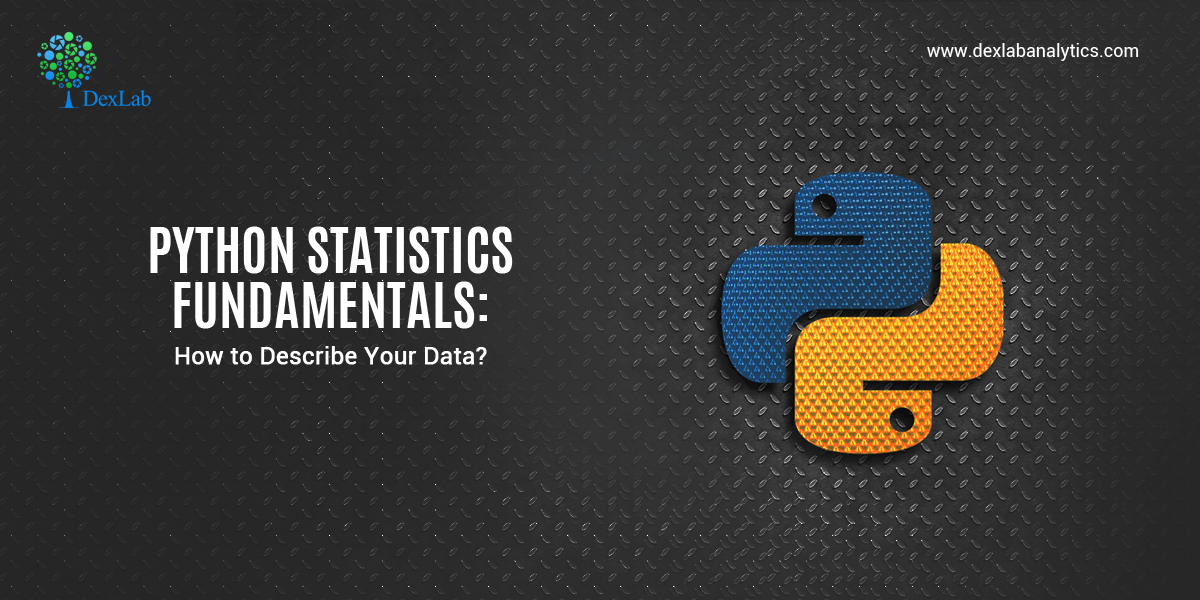Statistics is a branch of mathematics which deals with the collection, analysis, interpretation and presentation of masses of numerical data. Statistics is a tool used to communicate our understanding of data. It helps us understand the world better, make assertions, and communicate our confidence in the statements we are making.

#### Two main statistical methods are used in data analysis:

1. Descriptive statistics: This method is used to summarize data from a sample using measures such as the mean or standard deviation
2. Inferential statistics: With this method, you can conclude data that are subject to random variation (e.g., observational errors, sampling variation).

This whole topic will be covered in a series of two blogs. This first blog is about the types of measures in descriptive statistics. Furthermore, we will also see the built-in Python “Statistics” library, which has a relatively small number of the most important statistics functions.

Descriptive statistics can be defined as the measures that summarize a given data, and these measures can be broken down further into the measures of central tendency and the measures of dispersion. Measures of central tendency include mean, median, and the mode, while the measures of dispersion include standard deviation and variance.

#### We will cover the following topics in descriptive statistics:

• Measures of Central Tendency
1. Mean
2. Median
3. Mode
• Measures of Dispersion
1. Variation
2. Standard Deviation

First, we need to import the Python statistics module.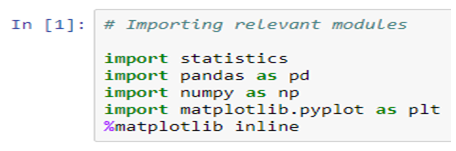#### Mean

The arithmetic mean is the sum of data divided by the number of data-points. It is a measure of the central location of data in a set of values that vary in range. In Python, we usually do this by dividing the sum of given numbers with the count of the number present. Python mean function can be used to calculate the mean/average of the given list of numbers. It returns the mean of the data set passed as parameters.

mean( ): Arithmetic mean (“average”) of data.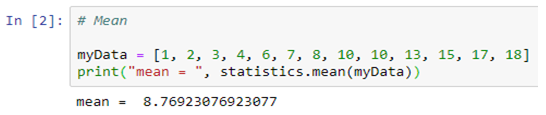harmonic_mean( ): It is the reciprocal of the arithmetic mean of the reciprocals of the data (say for three numbers a, b and c, 1/mean = 3/(1/a + 1/b + 1/c)).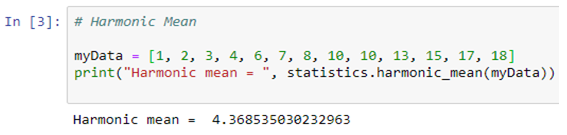#### Median

median( ): Median or middle value of data is calculated as the mean of middle two. When the number of data points is odd, the middle data point is returned. The median is a robust measure of a central location and is less affected by the presence of outliers in your data compared to the mean.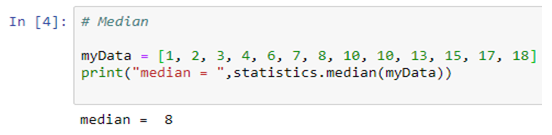median_low( ): Low median of data is calculated when the number of data points is odd. Here the middle value is usually returned. When it is even, the smaller of the two middle values is returned.

median_high( ): High median of data is calculated when the number of data points is odd. Here, the middle value is usually returned. When it is even, the larger of the two middle values is returned.

#### Mode

mode( ): Mode (most common value) of discrete data. The mode (when it exists) is the most typical value and is a robust measure of central location.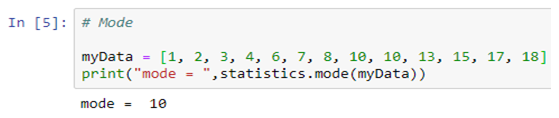#### Measures of Dispersion

Measures of dispersion are statistics that describe how data varies, usually relative to the typical value. While measures of centre give us an idea of the typical value, measures of spread give us a sense of how much the data tends to diverge from the typical value.

These following functions (from the statistics module in python) calculate a measure of how much the population or sample tends to deviate from the typical or average values.#### Population Variance

pvariance( ): Returns the population variance of data. Use this function to calculate the variance from the entire population. To estimate the variance from a sample, the variance ( ) function is usually a better choice. When called with the entire population, this gives the population variance σ². When called on a sample instead, this is the biased sample variance s², also known as variance with N degrees of freedom.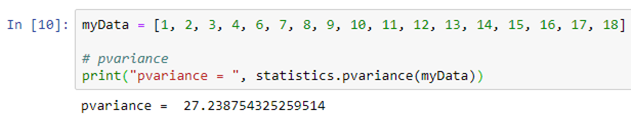#### Population Standard Deviation

pstdev( ): Return the population standard deviation (the square root of the population variance)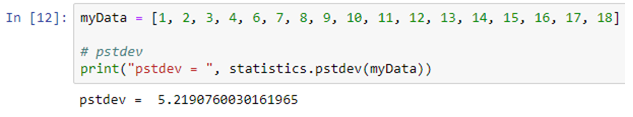#### Sample Variance

variance ( ): Returns the sample variance of data, an iterable of at least two real-valued numbers. Variance, or second moment about the mean, is a measure of the variability (spread or dispersion) of data. A large variance indicates that the data is spread out; a small variance indicates it is clustered closely around the mean. If the optional second argument is given to the function, it should be the mean of data. This is the sample variance s² with Bessel’s correction, also known as variance with N-1 degrees of freedom.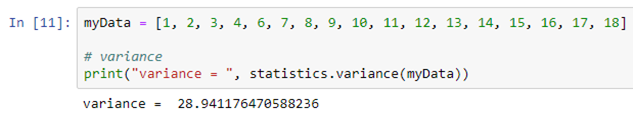#### Sample Standard Deviation

stdev( ): Returns the sample standard deviation (the square root of the sample variance)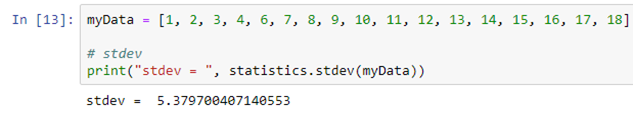#### Conclusion

So, this article focuses on describing and summarizing the datasets, also helping you to calculate numerical quantities in Python. It’s possible to get descriptive statistics with pure Python code, but that’s rarely necessary. In the next series of this blog we will see the Python statistics libraries which are comprehensive, popular, and widely used especially for this purpose.

## An All-Inclusive Guide on Python and its Changing Trends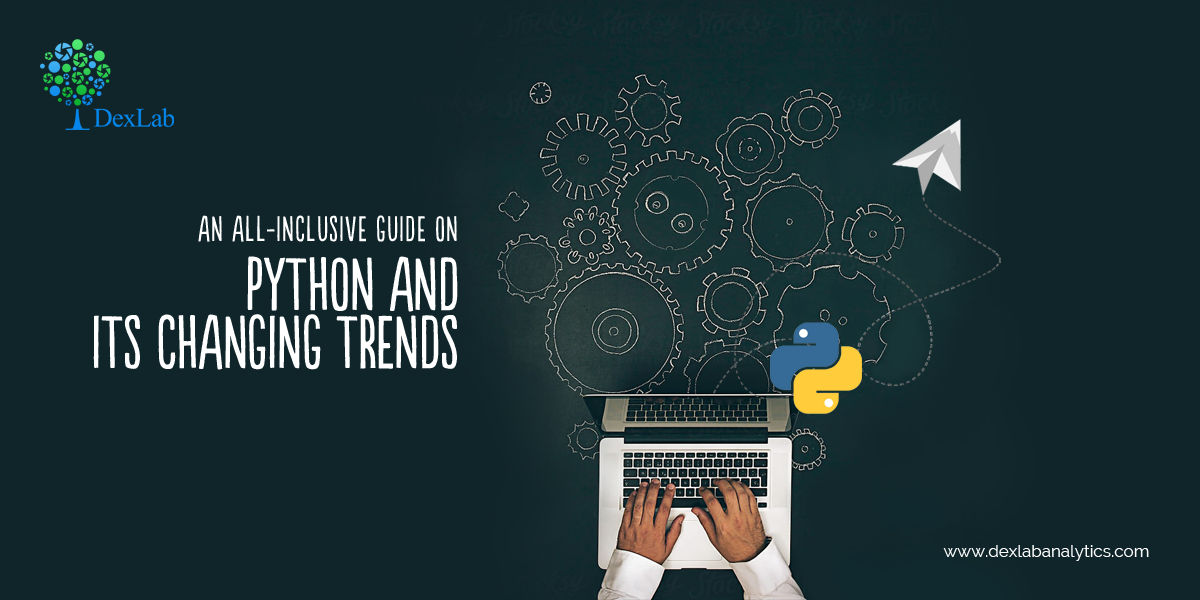Python is an extremely readable and versatile high-level programming language. Many companies such as Google, YouTube, Dropbox use the language for developing applications. It also finds its use extensively in diverse fields as in Python for data analysis, Machine Learning Using Python, Natural Language Processing, Web Development, Scientific Computing, Image processing, Robotics, Computer Vision and many more.

It supports both Object-oriented programming and Functional programming. Python is generally referred to as an interpreted language which implies that each line of code is executed one by one and if the interpreter finds an error, it stops immediately with an error message on the screen.

Another important feature of Python is its interactive prompt. A Python statement can be typed and immediately executed, which is in sharp contradiction to any other compiled language.

#### What are Python 2.x and Python 3.x?

There are two main versions of Python: Python 2.x and Python 3.x. If someone is new to Python, then he/she might be in confusion about which version to use. However, in the current scenario, we can easily migrate from Python 2 to Python 3, as the Python Software Foundation has finally taken the step to formally announce that Python 2 will reach the end of life (EOL) on January 1st, 2020.

#### Key differences between Python 2.x and Python 3.x

This article discusses the differences between these two versions of Python, making Python 3 less confusing for a new programmer.

1. #### Print Function

In Python 2, print is a statement. There is no need of parenthesis.In Python 3, print is a function. It needs parenthesis.1. #### Integer Division

In Python 2, if the division operator is performed on two integers, then the output will be an integer for example: – 7/3 = 2.In Python 3, if the division operator is performed on two integers, then the output will be accurate. It can also be in float for example: – 7/3 = 2.33.

To get the result in an integer only a different division operator is used that is (//) it returns an integer result for example, – 7//3 = 2.#### 3. Unicode Support

Both the versions of Python can handle strings (sequences of characters) differently.

Python 2 uses the ASCII encoding standard by default. ASCII is limited to representing 256 characters. This limits the flexibility of Python to encode the characters, particularly non-standard ones. Using Unicode in Python 2 requires extra syntax—for example when using print, the input text is to be wrapped in the Unicode() function to handle special characters.

In Python 3, Unicode is the default. The Unicode standard is much more versatile—it supports over 128,000 characters. There is no need for an extra syntax to define the Unicode values—they get printed automatically as utf-8 strings.

1. #### Range Function

In Python 2, the range function returns a list of numbers.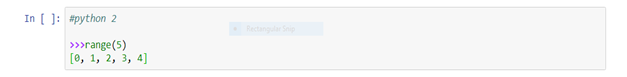In Python 2, the xrange class represents an iterable that provides the same object.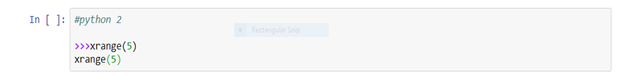In Python 3, original range function is removed and xrange is renamed to range: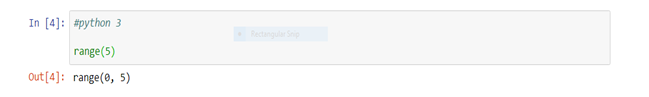In Python 3, it is needed to convert the range object to a list if someone desires the same result as the range function provides in Python 2.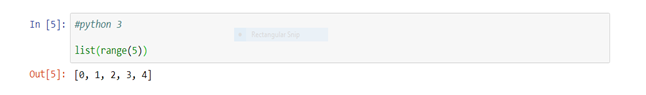1. #### ­­­­Input() Method

Mainly what is expected from the input() method is that it reads input as string, then it can be converted into any datatype as per the requirement.

In Python 2, it has both the input() and raw_input() methods for taking input. The difference between the raw_input() and input()is that the raw_input() reads input as a string while the input() reads input as string only if it is inside quotes else reads as an integer.

In Python 3, there is no raw_input() method. The raw_input() method is replaced by input() in python 3.

If someone still wants to use the input() method like in python 2, then it can be availed by using eval() method.

1. #### Next() Method

In Python 2, .next() method is used and in Python 3 next() function is used to iterate the next element of an iterator.

1. #### Raising Exception

To raise an exception in Python 3, the argument should be in parenthesis, while in Python 2, it is not necessary.

1. #### Handling Exception

Handling exception is also changed in Python 3, “as” keyword is used in Python 3, while it is not necessary in Python 2.

So, if someone is a beginner, then it is strongly recommended to use Python 3 because it is the future of Python and also January 1, 2020, will be the last day of Python 2. It means that no improvement will be done anymore after that day, even if someone finds a security problem in it.It is highly recommended to upgrade the version of the programming language to Python 3. Some ways can help the Python 2 users in porting their code from Python 2 to Python 3 and get the feel of Python 3 and figure out how it is different from Python 2. The code can be imported by using tools like “Futurize” and “Modernize”. Also, if someone wants to check the availability of Python 3 as part of his tests, then “caniusepython3.check()” can be used.

As a final note, everyone must look for upgrading their Python version to Python 3 to understand the subtleties of the new version and usher in the future. However, if you are interested in Deep learning for computer vision with Python and similar courses, then opt for the premium Python training institute in Delhi now!

.

## Statistical Application in R & Python: Poisson Distribution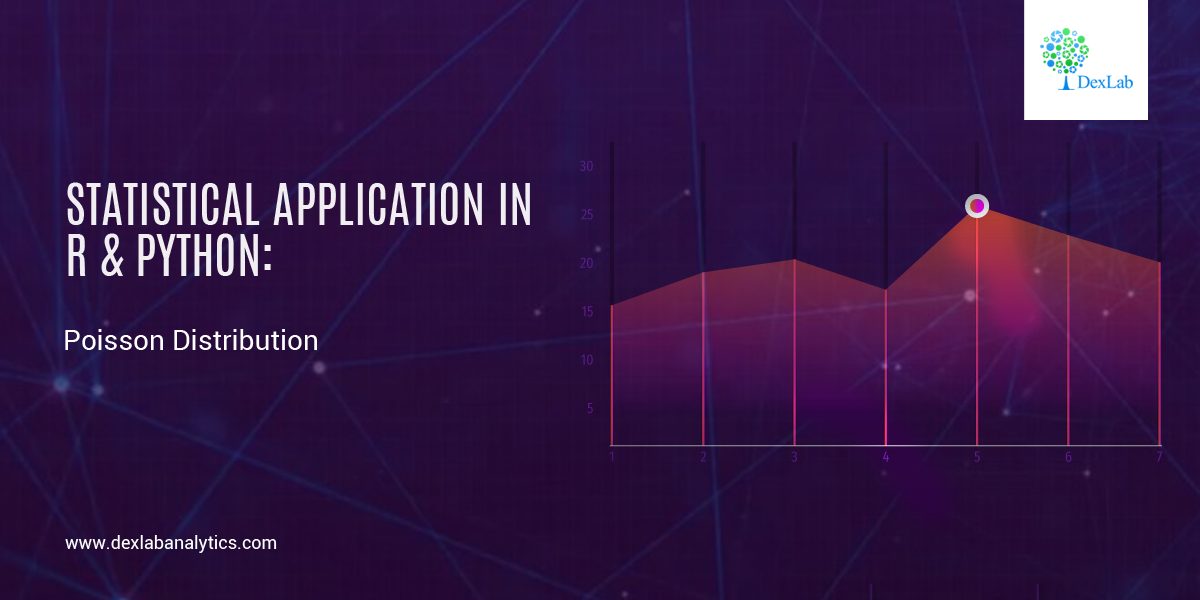Continuing with the series of blogs, the first of which was Statistical Application In R & Python: Normal Probability Distribution, here we bring you a post on how you can calculate Poisson distribution effortless using R & Python. So, stay tuned!

Poisson distribution is a counting process which is a discrete probabilistic model. It has only one parameter, (lambda or “m”) which is essentially the average rate of change. Poisson distribution is used to model “number of anything”. The probability distribution function of a Poisson distribution is given by the below expression.

If m is the mean occurrence per interval, then the probability of having x occurrence with in a given interval is: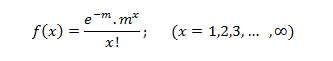#### Application:

A business firm receives on an average 6.5 telephone calls per day during the time period 11:00 – 11:15 A.M., Find the probability that on a certain day, the firm receives exactly9 calls during the same period.

The random variable x is the ‘number of telephone calls received during the period 11:00 – 11:15 A.M, since x is assumed to Poisson distribution. The parameter m is equal to the mean of the distribution; i.e.  m = 6.5 and x = 9, then the equation is: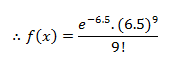#### Calculate Poisson Distribution in R: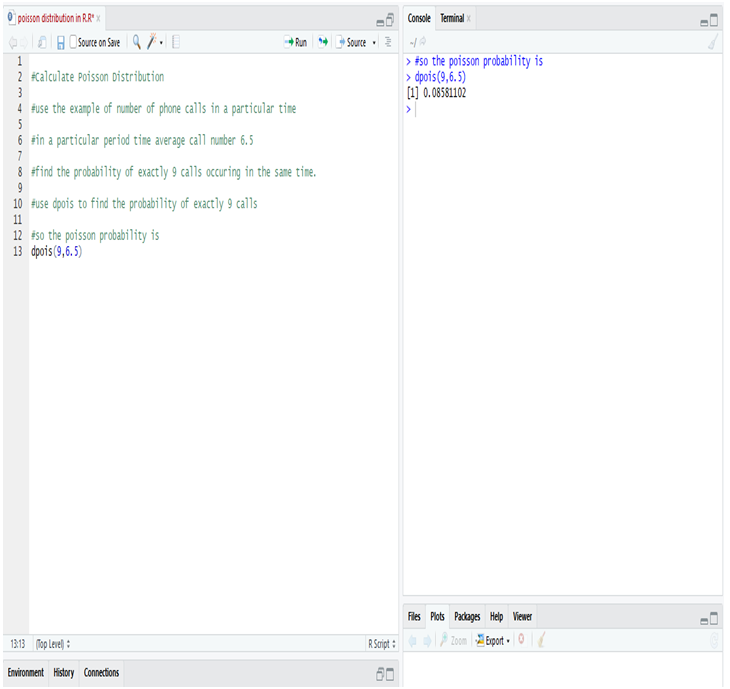So, while calculating Poisson distribution in R, we notice that the probability of occurring exactly 9 calls instead of average 6.5 calls in a given particular time (11:00 A.M – 11:15 A.M ) = 85.81%

#### Calculate Poisson Distribution in Python: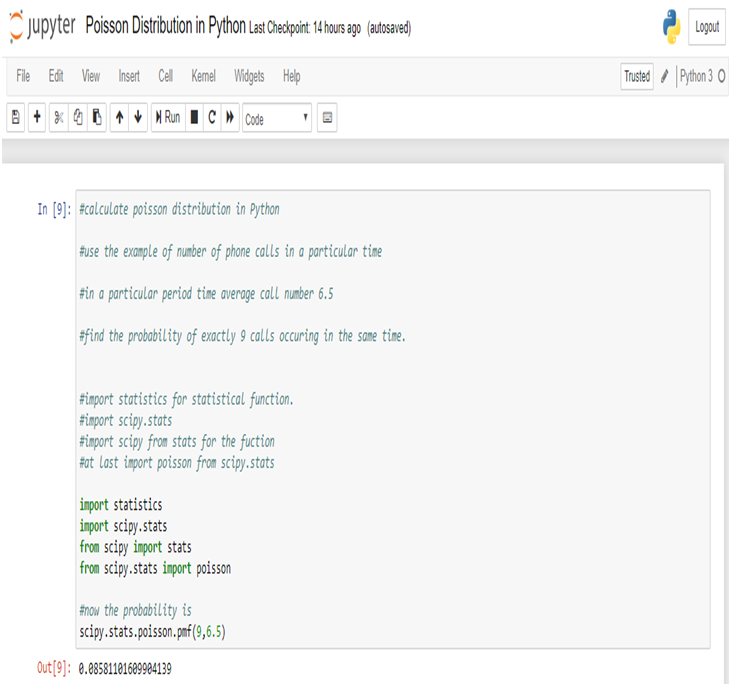So, while we calculate Poisson distribution in Python, we notice that the probability of occurring exactly 9 calls instead of average 6.5 calls in a given particular time (11:00 A.M – 11:15 A.M) = 85.81%

#### Conclusion:

Companies can use the Poisson distribution to contrive effective steps to improve their operational efficiency. For instance, an analysis done with the Poisson distribution might reveal how a company can arrange staffing in order to be able to handle the peak periods efficiently, when the customer service calls keep on pouring.

In this problem we see that the business firm receives on an average 6.5 telephone calls per day during the time period 11:00A.M – 11:15A.M, then the probability of the firm receives exactly 9 calls in a same is 85.81%.

Dexlab Analytics is the best Python training institute in Delhi, bringing you the all-inclusive courses of Python for Data Analysis and R Predictive Modelling Certification, among others to start your career in Data Science and Analytics.

## A Nifty Guide to Initiate AIOps in 2019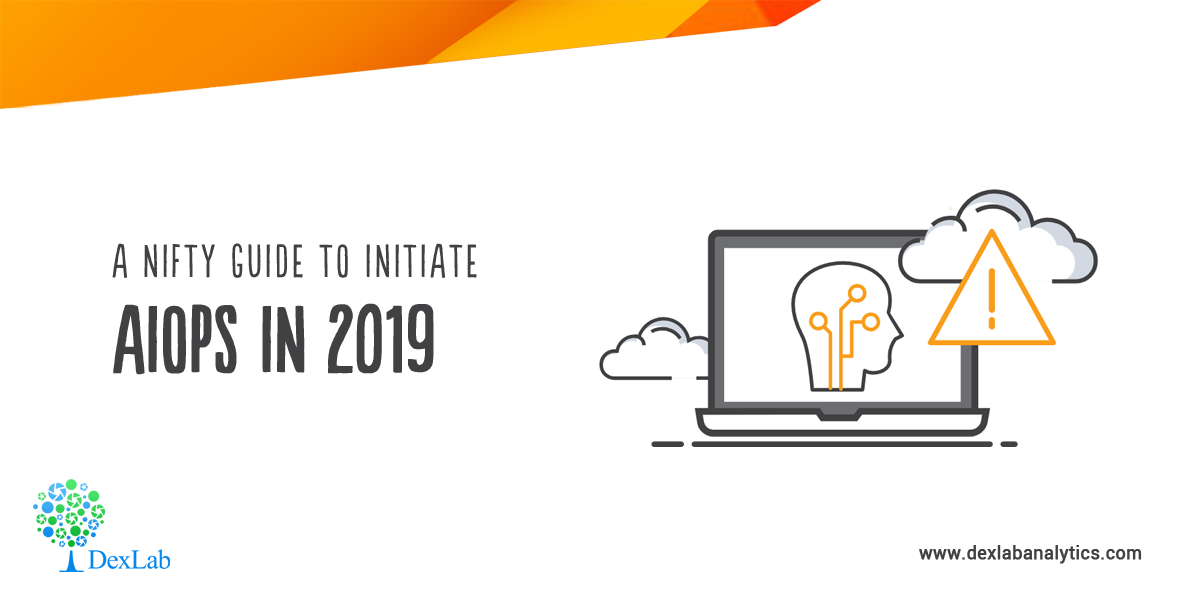AIOps (artificial intelligence for IT operations) is the buzz word of the 21st century.

In this digitally-charged world, AIOps platforms are the key. They fuse ML and big data functionalities to boost and partly replace primary IT operations’ programs, including event correlation and analysis, performance monitoring and IT service automation and management.

In simple terms, AIOps is the combined application of data science and machine learning to help mitigate IT operations-related challenges and find faster insights. It fixes high-severity outages in a jiffy.

The main objective of revolutionary AIOps platforms is to ingest and analyze the aggravating volume, variety and velocity of data and deliver it in a useful manner.IT bigwigs are excited about the prospects of applying AI and ML to IT operations.

Gartner expects that big enterprises’ usage of AIOps and other monitoring tools and applications will rise from 5% in 2018 to 30% in 2023. The long-term impact of AIOps on IT operations is predicted to be transformative.

Fortunately, AI capabilities are making headway, and more real-time solutions are being formulated and made available each day.

Read on to know how to get started with AIOPs:

#### Be prepared

First and foremost, you have to familiarize yourself with all the ML and AI capabilities and vocabulary. It doesn’t matter if you are gearing up for an AIOps project or not. Capabilities and priorities change; so be ready to implement the platform anytime soon.

#### Select the first few test cases carefully

Small and steady wins the race. The same phrase applies to transformation initiatives. They start small, seize knowledge and iterate from there. Imbibe the same approach for AIOps success.

Decode the intricacies of AIOps amongst your colleagues by displaying simple techniques. Ascertain your skills and identify the loopholes, then devise a relevant plan to fill up those gaps in-between.

#### Feel free to experiment

Although a majority of AIOps platforms are complex and costly, there is a substantial number of open-source and relatively low-cost ML software available in the market that lets you evaluate the efficacy of AIOps and ML applications and their uses.

#### Look beyond IT

Don’t forget to leverage all kinds of data analytics resources available in your organization. Data management is the cornerstone of AIOps. Most of the teams are already skilled in it. Statistical analytics and business analysis are key components of contemporary business frameworks, and many techniques traverse public domains.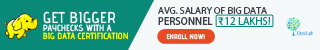#### Standardize and modernize, as and when required

Prepare your work infrastructure to implement a robust AIOps adoption by embracing secure automation architecture, immutable infrastructure patterns and infrastructure as code (IaC).

Interested in learning more about Machine Learning Using Python? Feel free to reach us at DexLab Analytics. We’re a premier learning platform specialized in offering in-demand skill training courses to the interested candidates.

The blog has been sourced from ― www.gartner.com/smarterwithgartner/how-to-get-started-with-aiops

## Statistical Application in R & Python: Normal Probability Distribution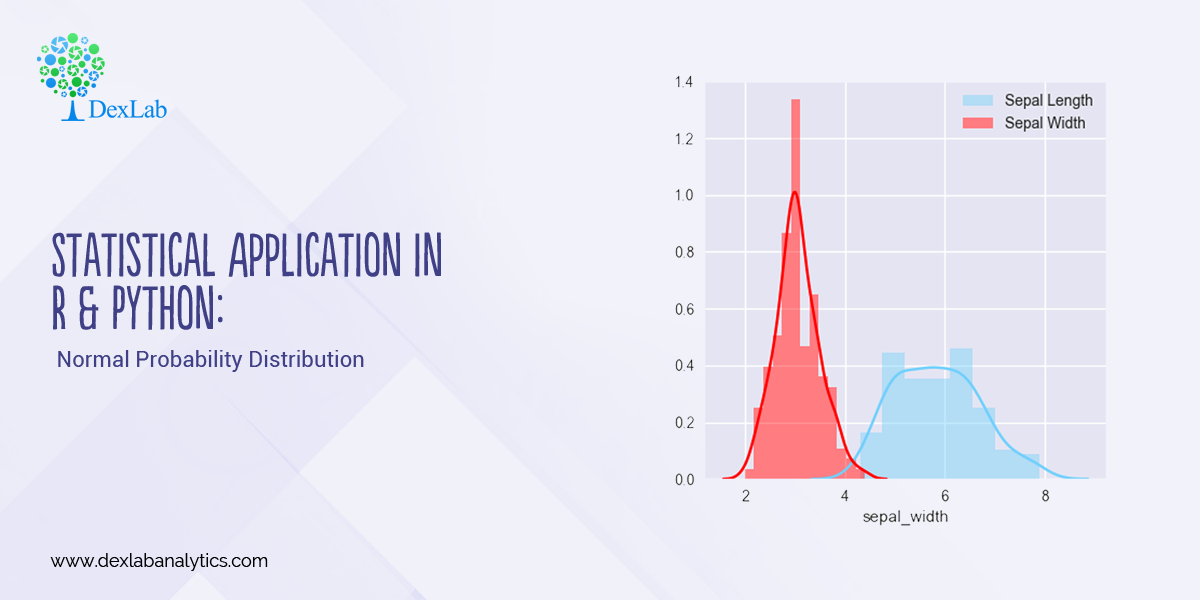Gauss, the famous French Mathematician is responsible for developing one of the most significant distributions in all of statistics, i.e. – The Normal Distribution. Please refer to the blog on Central Limit Theorem: www.dexlabanalytics.com/blog/the-almighty-central-limit-theorem. It will help you fully grasp the significance of the Normal Distribution. However, if you want to revisit our series of blogs by following it from the start, you can reach STATISTICAL APPLICATION IN R & PYTHON: CHAPTER 1 – MEASURE OF CENTRAL TENDENCY right now!

Essentially, the Normal Distribution provides “approximations” to most other distributions such as the Binomial, Poisson, Gamma, Exponential, etc. This is to say as sample sizes get statistically large enough, most distributions approximate into a normal shaped curve.

Every distribution has important features known as its “parameters”. Normal distribution has two parameters. These are Mean ( ) and Variance (σ²). The normal distribution has a bell-shaped curve, where the probability of likelihood peaks at its mean in the middle.

The Normal Distribution has vast practical applications in the field of Business, Finance, Medicine, and Physics and so on. Things like weights, heights, IQ scores follow the Normal Distribution.

Normal Distribution, Gaussian distribution, is a continuous probability distribution and is defined by the Probability Density Function (PDF).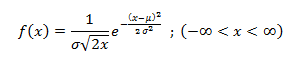Where,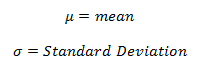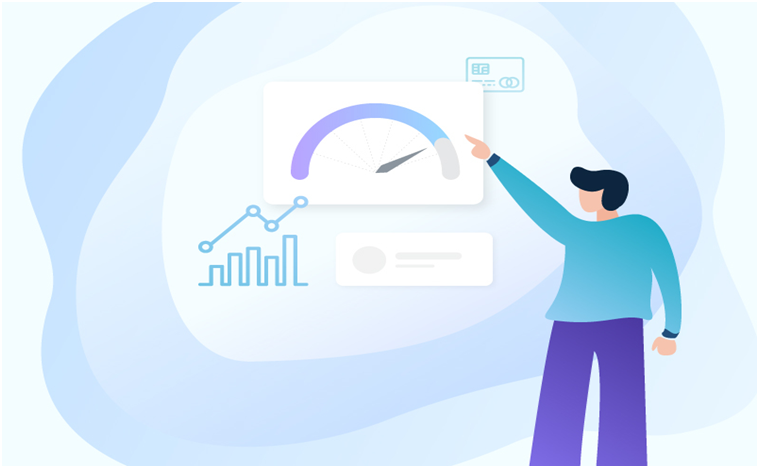#### Application:

Assume that the credit score fits a Normal Distribution.

Suppose Mr. Arjun’s last 10 month’s credit score are:

789, 635, 739, 687, 724, 810, 817, 735, 819, 820

What is the probability that the percentage of credit score will 825 or more in the 11th month?

 Months Credit Score January 789 February 635 March 739 April 687 May 724 June 810 July 817 August 735 September 819 October 820#### Calculating Normal Distribution in R: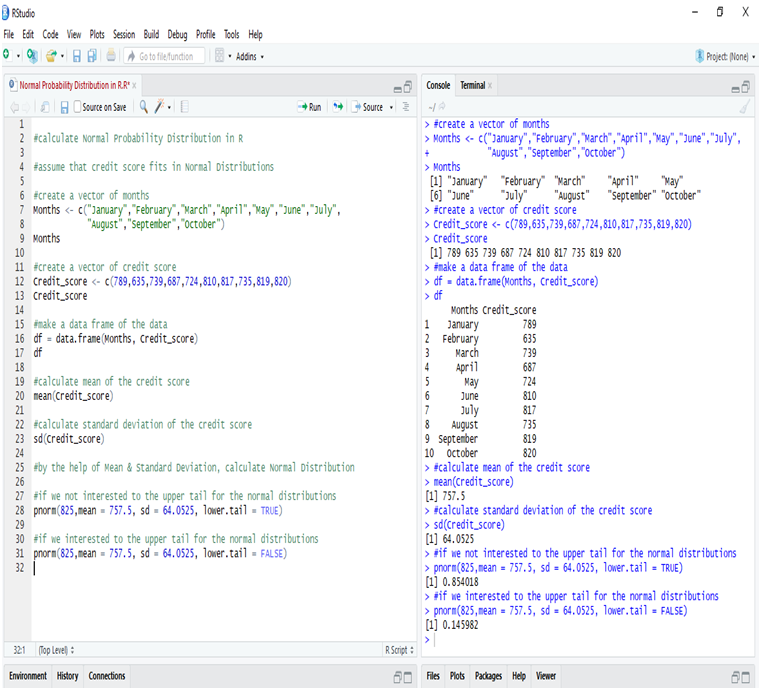If we go to calculate Normal Probability Distribution in R, we can predict that the probability of the 11th month credit score will be 825 or greater than that is 14.60%, whereas in another case, the probability of the 11th month credit score will be 825 or less than that is 85.40%.

#### Calculate Normal Distribution in Python:

Make a data frame of the data and calculate Mean and Standard Deviation for calculate Normal Distribution.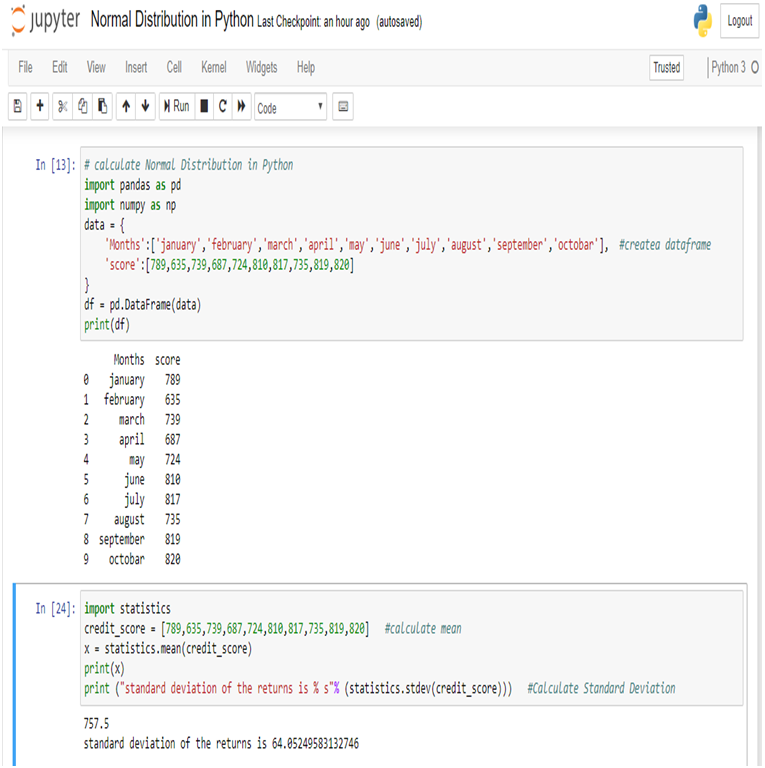Now, we can easily calculate Normal Distribution in Python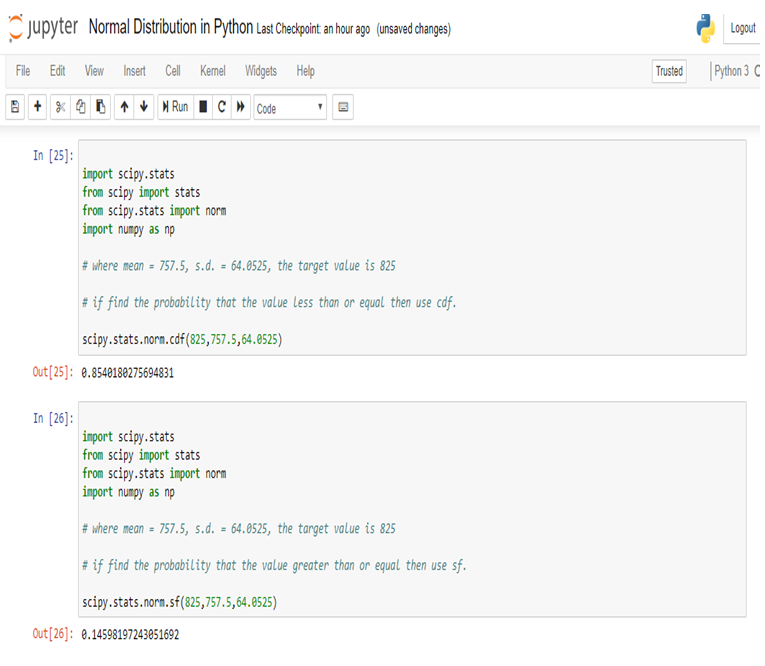So, in calculating the Normal Probability Distribution in Python, we can predict that the probability of the 11th month credit score will be 825 or greater than that is 14.60%, whereas in another case, the probability of the 11th month credit score will be 825 or less than that is 85.40%.

#### Conclusion:

Normal Distribution is used for calculating parameters. It is represented by the bell curve, where the total area of the curve is 1. Normal Distribution has its use in Finance, Business, Salaries, Blood Pressures, Measurement etc and many other fields.

Here, we have used Normal Distribution to predict Mr. Arjun’s 11th month credit score, and set the target (825). By Normal Distribution we can predict the percentage of possibility to achieve the target.

Calculating Binomial Distribution might be tricky for many but with Dexlab Analytics it won’t be hassle anymore. So, get hold of our STATISTICAL APPLICATION IN R AND PYTHON: CALCULATING BINOMIAL DISTRIBUTION blog, to get around all your problems.

## Statistical Application in R and Python: Calculating Binomial Distribution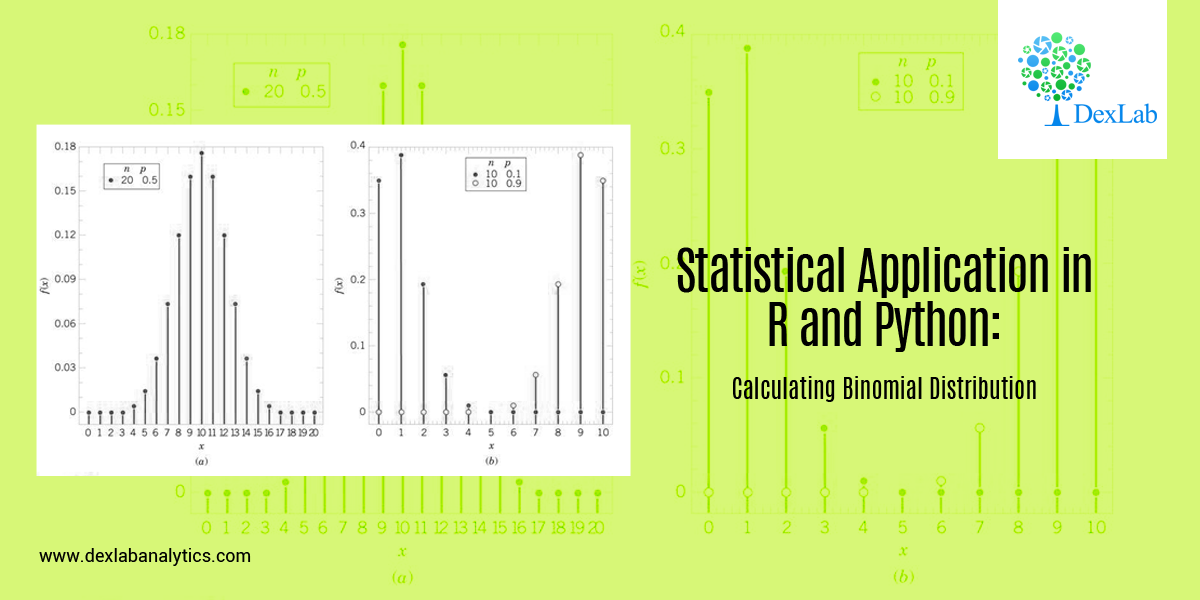In this blog, we will take a look at the Binomial distribution. This blog is among the series of blogs through which you’ll have a vivid idea of the Statistical Application using R and Python. Statistical Application In R & Python: Chapter 1 – Measure Of Central Tendency is the first of such blogs.

The binomial distribution is an extension of the Bernoulli distribution. In Bernoulli, we have only one parameter, i.e. the probability of success.

Now, consider a case where we have “n” number of trials and we want to predict the probability of success from it. This is the Binomial case.

Binomial distribution has two parameters, i.e.: number of trails (n) AND probability of success (p). The mean of the binomial is a product of its two parameters, i.e. n multiplied by p. It is a discrete probability distribution. Here, each trial is assumed to have only two outcomes, either success or failure.

If X be a discrete random variable (taking only non-negative values), it is said to be following binomial distributions with a probability mass function as:-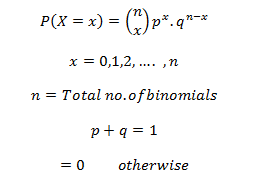#### Application:

A food shop starts a offer for a festive season, They have 12 different baskets, each basket has 5 combos and only 1 of them is non-veg. Find the probability of having 4 or less non-veg combos, if a consumer tries every combos at random.

Since, only 1 out of 5 combos is non-veg, the probability of choose a non-veg combos by random is 1/5 = 0.2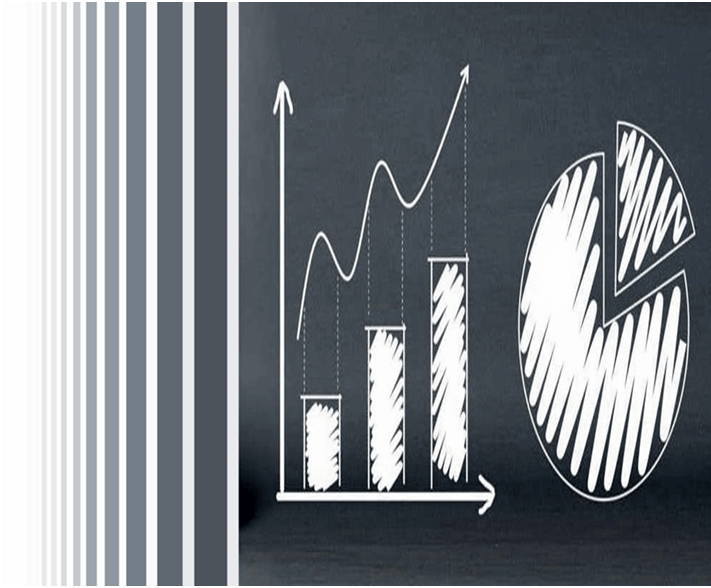#### Calculate Binomial Distribution in R: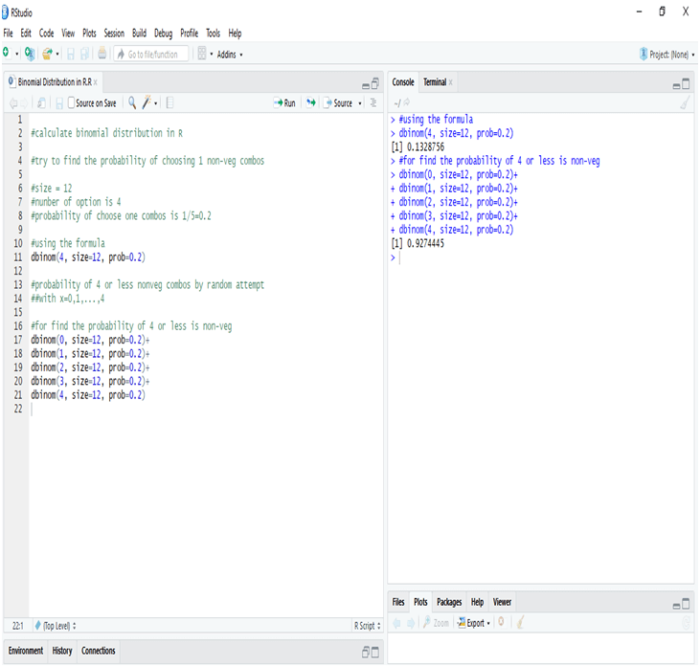In R the probability of one non-veg combos choose by random in 5 is 13.28%, whereas the probability of four or less combos choose by random in a twelve baskets is 92.44%

#### Calculate Binomial Distribution in Python: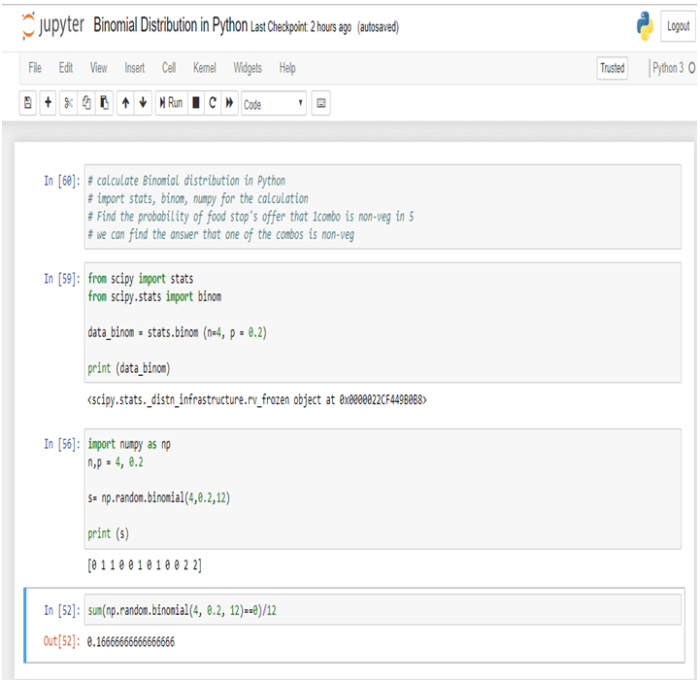In Python the probability of one non-veg combos choose by random in 5 is 16.66%.

#### Conclusion:-

Binomial Distribution is the process by which we can calculate the probability of success from “n” number of trails. In Binomial Distribution we can find only two outcomes like “Yes” or “No”.

Dexlab Analytics is a pioneering institute of Data Science, with peerless trainers to help you ease your journey with Python Certification, R Programming Certification and Big Data Certification along with numerous other advanced and/or career oriented courses in Computer Science.

## Hacking is Wide and Dangerous in India, CBI ReportsThe recent conference organized by the Central Bureau of Investigation on Cyber forensic notes that over 22,000 websites were hacked in India between April 2017 – Jan 2018. Not the best of the news for the nation which is largely counting on their citizens to be tech-savvy.

In the conference, CBI disclosed of its plans to build a cutting edge Centralised Technology Vertical (CTV) to fight crimes, voiced by Minister of State for Personnel, Jitendra Singh. The CTV is a huge project involving around Rs 99 crore, which will not only share the real-time information about the cyber attacks but also of the perpetrators.

From young superintendents of police to top brass of security agencies, police forces, law enforcement officers and the Intelligence attended this conference and discussed about the alarming rise of cybercrimes throughout the country.#### The Major Issue

Jurisdictional issues were a main problem and hit greatly on the investigation in these cases because most of the incidents of cybercrimes are triggered from foreign lands. Though the total loss of money from the recent cybercrimes weren’t disclosed, some debilitating cases in cybercrimes were dicussed once again, which included the loss of USD 171 million from union Bank of India’s Swift.

#### To End it

To lessen the magnitude of the cybercrimes, the CBI is on their way towards reinforcing them with the state of the art technology. Besides, you can also take up courses in PHP, HTML, Python Certification Training in Delhi, to be informed of the trending languages and be future proof.

## Take a Deep Look on How Machine Learning Boosts Business Growth!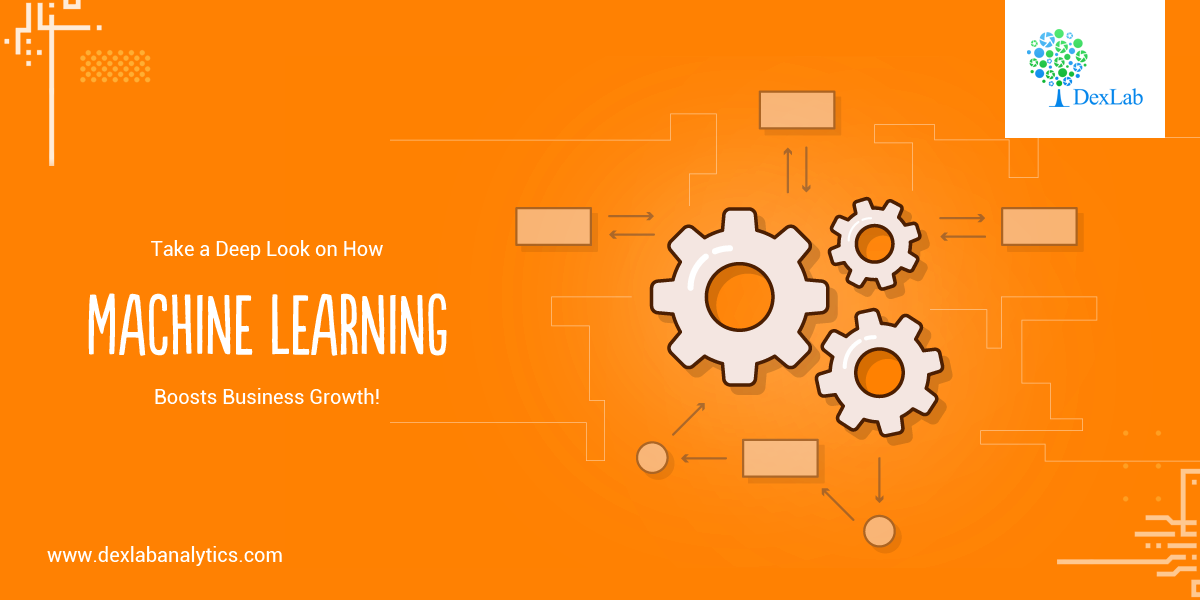Machine Learning is the technology of the future and the rise of it is, well, shocking! Numerous businesses have already started adopting Machine Learning into their business strategy which is ultimately culminating towards their growth. You can also get the most of Machine Learning by going for the best Machine Learning course in India without wasting hours on the internet.

This new and improving technology is showing marked results in making a particular business more efficient, enhancing customer relationships and driving more sales than ever. You can get right on to Machine Learning Significantly Aids in Improving the Business Performance: Learn the Hows and learn about Machine Learning and its rising curve.

Here we have decided to discuss in details about the ways how Machine Learning is helping business touch great heights:#### Natural Language

One of the major setbacks in the industry of computer science was the inability of computers to comprehend our natural language or the way we speak in our everyday life. This is slowly changing with the rapid growth and considerable research and development on Machine Learning.

It looks like we have come a long way from the crude search terms that we used to generate the results that we wanted. The AI-driven programs of now, with the help of Machine Learning, can figure out the essence of our conversations and also capitalizing largely on the nuances of our language. Most importantly, they learn from past experiences, which is highly progressive.

#### Logistics

The retail industry and that of logistics are largely relying on Python for Data Analysis and this in turn, is making them future-proof.

Retail giants like Amazon are encouraging the use of Machine Learning to sharpen the efficiency of their company with new features and technology like “anticipatory shopping” protocol. Retail analytics using Python is becoming formidable.

Even in the field of logistics, the inclusion of Machine Learning is proving a boon!

#### Manufacturing Industry

Innumerable manufacturing companies are adopting the budding technology of Machine Learning and utilizing it in almost every stage of production, simply because the AI-driven technology reduces unnecessary expenses.

Companies like Seebo, are taking up Python seriously to build accurate data analytics software. Moreover, machine learning is estimated to cut down on the delivery times by 30% and surprisingly save fuel by 12%. According to the reports, the programs fed on AI would even reduce the maintenance costs by 20 – 30%.#### Consumer Data

We have already seen a world of data collection which has been on a rise for years. Now, finally, with the rise of machine learning, the companies are looking forward to making some use of all these data that they have accumulated. In the coming years, we will see AI improving powered by Machine Learning to make the world productive and smart all the more.

You can take a look at A DISCUSSION ABOUT ARTIFICIAL INTELLIGENCE: KNOWING AI CLOSELY if you are interested in AI. Stay glued to our website for more updates and information from the world of technology!

## Application of Median Using R And Python: Calculating Median On the Go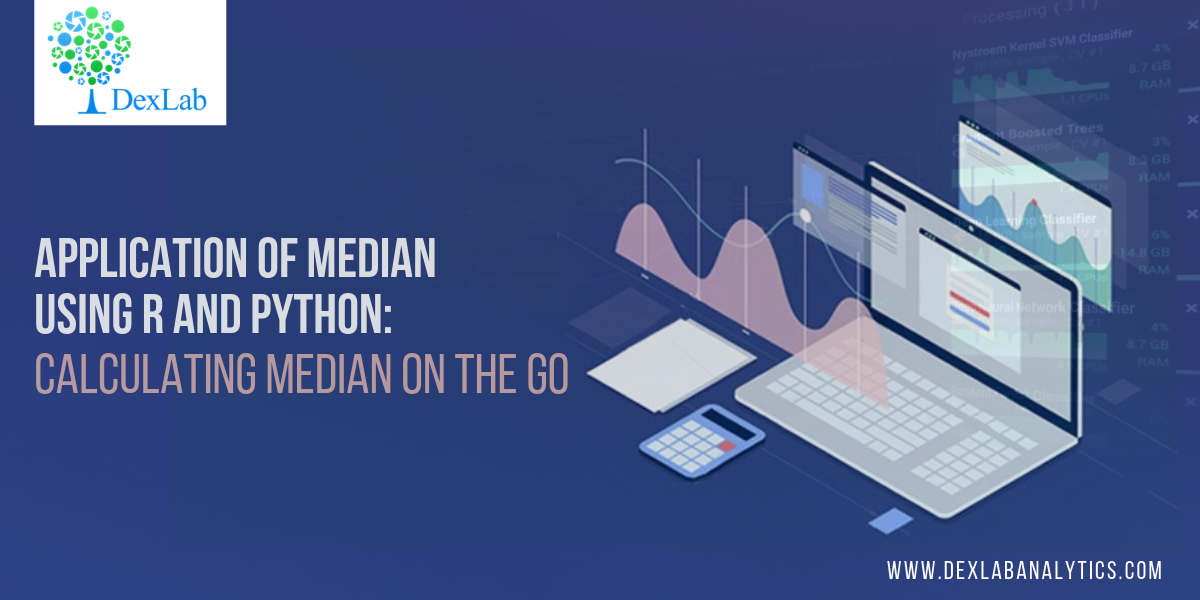This blog is in continuation of STATISTICAL APPLICATION IN R & PYTHON: CHAPTER 1 – MEASURE OF CENTRAL TENDENCY and takes you through a comprehensive way to calculate the Median in R and Python.

The term ‘Median’ is derived from the Latin word – ‘Medius’ means the center of something. In mathematics, Median is treated is that unique observation which would divide your data set into two equal halves.

If you are still unclear about Mean and/or seeking easier ways to calculate Mean using R & Python, then check

Median is special because unlike its rival, the Mean, Median is not ridiculed by the curse of extreme values. To illustrate the curse of extreme values, we bring you the following example:

In Lacs

##### 36

The mean of the above data set is: 88/7 = 12.57 lacs.

Whereas, to get the median we would have to first arrange the data into ascending order and look for the midpoint of my data i.e.,(1/2 + n/2)th observation. Where “n” is the number of observations.

The median would then be:

##### 36

Median is the 4th observation, which is 8.5 lacs.

Looking at the mean and median, it would be fair to conclude that median is the better choice to accurate summarizing the data set whenever extreme values are present. However, this may be a crude generalization which should be taken with a pinch of salt. Despite its flaws, the mean still has statistical properties used in predictive analytics which the median lacks.#### Application:

A construction company gave wages to their 10 labor (Let name A to J)  as a weekly basis, the wages are 2000, 2100, 1900, 2150, 2500, 2450, 1800, 2600, 2200, 2300. Compute the Median wages of the construction company.

Sr.NoLaborsWages (Weekly)
1A2000
2B2100
3C1900
4D2150
5E2500
6F2450
7G1800
8H2600
9I2200
10J2300

#### Calculation Median in R: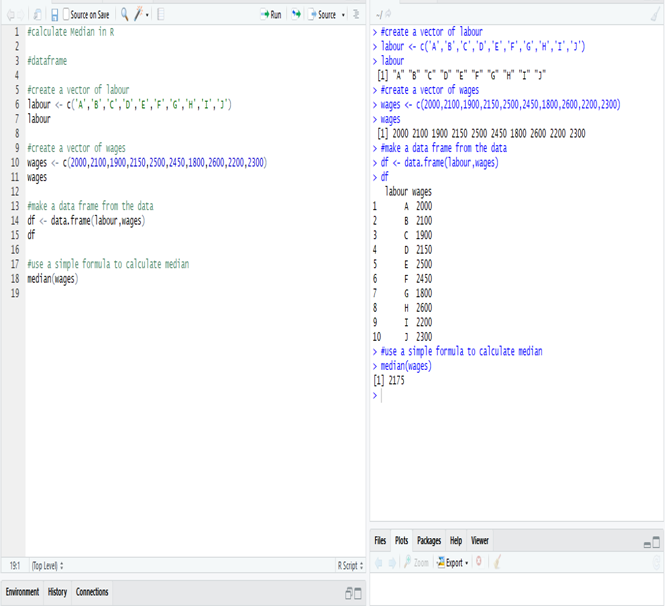The Median wage is 2175, calculate in R.

#### Calculate Median in Python:

Create a data frame of the data in Python.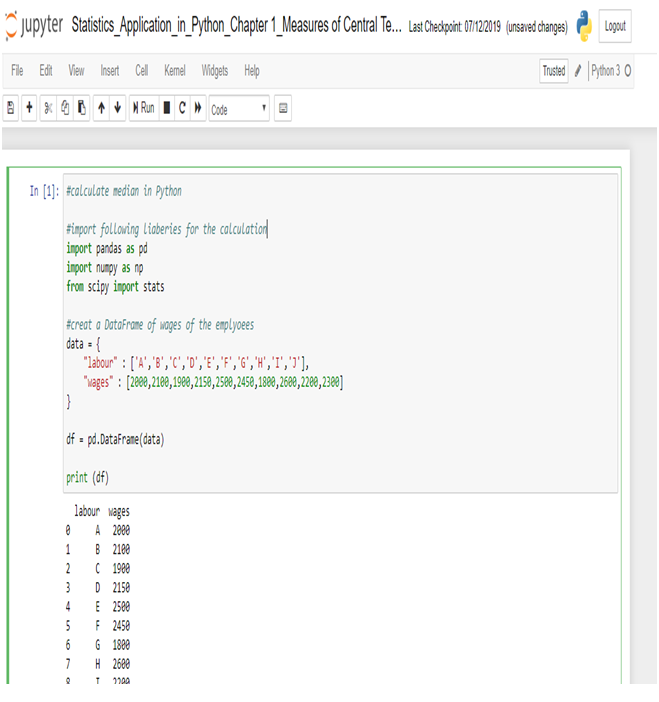Now, calculate Median in Python.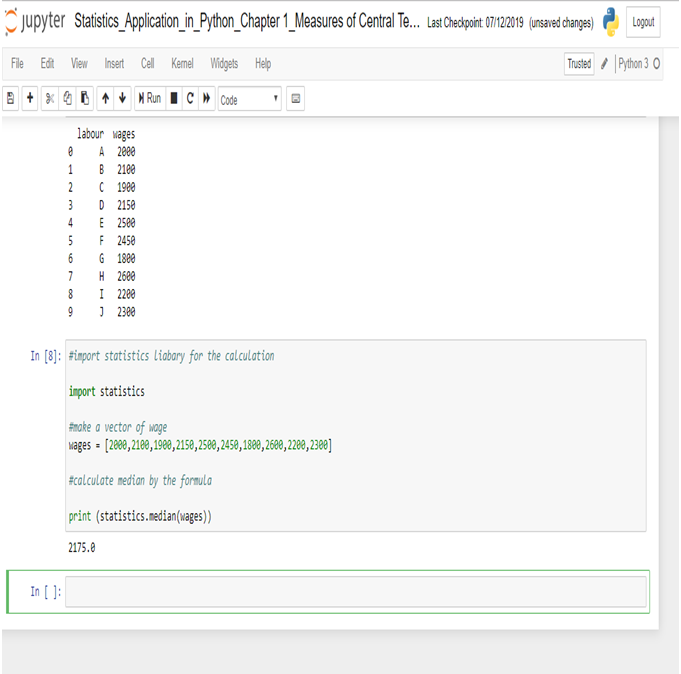The Median wage is 2175, calculated in R.

This concludes the post. If you have any queries with regards to this post, you can reach us at Dexlab Analytics. Furthermore, you can also look up for interesting and quality courses of R Programming Certification, Python Certification. Also, you can enroll with us for our combined courses of Data Science with Python Certification, Deep Learning and AI using Python, among others. So, hurry up and grab the best course!